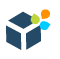DWBI.org Login / Sign Up
New Account?
Recovery
SAP Data Services

# SAP Data Services Analytic Functions

In this tutorial we will learn how to implement Cumulative Sum in SAP Data Services (BODS). Since there is no available in-built Analytic Functions in SAP Data Services, we will achieve the end result by exploiting some in-built Data Services features. Also this tutorial will show us how to manipulate Data Flow Parameter values at Data Flow level itself by using the trick of Custom Function calls.

### Implementing Running Sum partitioned by department

Let us consider the case where we want to calculate the cumulative sum of employee salary for each department. In other words, we are trying to calculate the sum of salaries of the input employee records read so far partitioned by department. Unlike a simple running sum, here we need to reset the sum to zero everytime a new department is encountered. To be able to do this, we must access the values of the previous record while processing the next record.

#### Setting up the Batch Job

So let us build a Batch Job as shown below. For the cumulative sum implementation two Data Flow Parameters are defined at the dataflow level. Click on the Variables and Parameters button in the tool bar and go to Calls tab. Next initialize these dataflow parameters \$PREV_SAL and \$PREV_DEPT to 0.00 and 0 respectively.

#### Creating the Data Flow

Below picture shows the implementation Data Flow. We will go through each of these Transforms separately.

At Dataflow workspace, click on the Variables and Parameters button in the tool bar again and go to Definitions tab. Next define two Data Flow Parameters \$PREV_SAL of Data type decimal(7,2) and \$PREV_DEPT int with Parameter type Input. These are the parameters which will store the values from the previous record. After we define the dataflow parameters, then only we can initialize their values at their Parent Job or Workflow level.

The previous screenshot describes the same.

In the Query Transform we select the columns of interest namely DEPTNO and SAL from EMP table as the Schema-Out. The data is being sorted with respect to DEPTNO and SAL in ascending order of value. This will ensure records belonging to one department will always be adjacent.

#### Create a Custom Function

Let us create a new Custom Function in the Local Object Library namely "CF_CHECK_DEPT_SAL" and create some Parameters for that.

 Parameters Data type Parameter type Return int Output \$CURR_DEPT int Input \$CURR_SAL decimal(7,2) Input \$PREV_DEPT int Input/Output \$PREV_SAL decimal(7,2) Input/Output

We will pass the Current row value and the Previous row value as Input Parameters to the function, so that we can decide what to do in each case.

Concretely, we will compare the current department value with the previous department value to check whether we are still in the same or a different department.

For the same department group, we will increase the cumulative sum by the current salary.

#### Parameter Short-circuiting

Here within the function, we basically set the \$PREV_DEPT, \$PREV_SAL Parameters of type Input/Output to something and since it is of type Input/Output the changed values is passed back into the Dataflow Parameters. So by using Custom Function we can modify and pass values for a Dataflow Parameter. Hence these two parameters defined at Dataflow level is short-circuited with the two Input/Output parameters of the Custom Function.

``````if ( \$CURR_DEPT = \$PREV_DEPT )
\$PREV_SAL = \$PREV_SAL + nvl( \$CURR_SAL, 0.00 );
else
\$PREV_SAL = nvl( \$CURR_SAL, 0.0 );

\$PREV_DEPT = \$CURR_DEPT;
Return 0;``````

#### Calling the Custom Function

In the next Query Transform, select DEPTNO and SAL from Schema-In as a part of Schema-Out. Aditionally right-click on the Schema-Out, create a New Function Call and select the Custom Function namely "CF_CHECK_DEPT_SAL".

Next select the input argument values.

The current value gets the value from the Schema-In of the query transform, while the previous value was stored in the parameter variable.

Click Next and select the output function parameters. Select the \$PREV_SAL parameter as output, having the cumulative salary for the current row.

Finally we format the output schema and map the result to a flat file format.

This is how the input and the desired output dataset looks like.

### Aggregation without using GROUP BY clause

Next with the cumulative salary sum already in place let us try to find the total salary of each department. Simply we can get the result by using the group by clause for the source data. But lets play with the reult set we have at the Schema-Out of the Query transform namely QRY_CUST_FUNC.

First we will sort the data based on Department number ascending and Cumulative Salary in descending order. So at the end of this transform for first unique row for each department will basically have the total salary for that department.